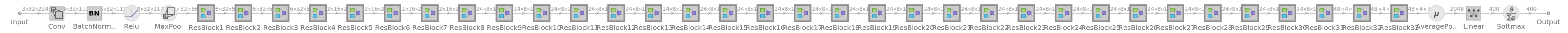# Channel-Separated Video Action Classification Net Trained onKinetics-400 Data

Identify the main action in a video

Inspired by 2D separable convolutions in image classification, the authors propose 3D Channel-Separated Networks (CSNs), in which all convolutional operations are separated into either pointwise 1×1×1 or depthwise 3×3×3 convolutions, resulting in a significant accuracy improvement on Sports-1M, Kinetics and Something-Something datasets while being two to three times faster.

## Training Set Information

• Kinetics-400 human action video data, consisting of four hundred human action classes, with at least four hundred video clips for each action. Each clip lasts around 10 seconds and is taken from a different YouTube video.

## Model Information

•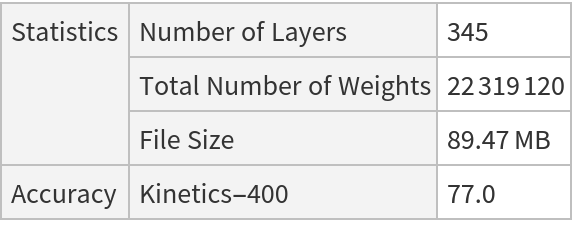## Examples

### Resource retrieval

Get the pre-trained net:

 In:=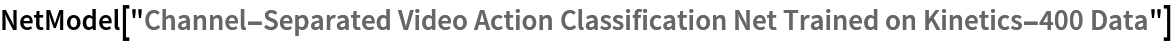Out=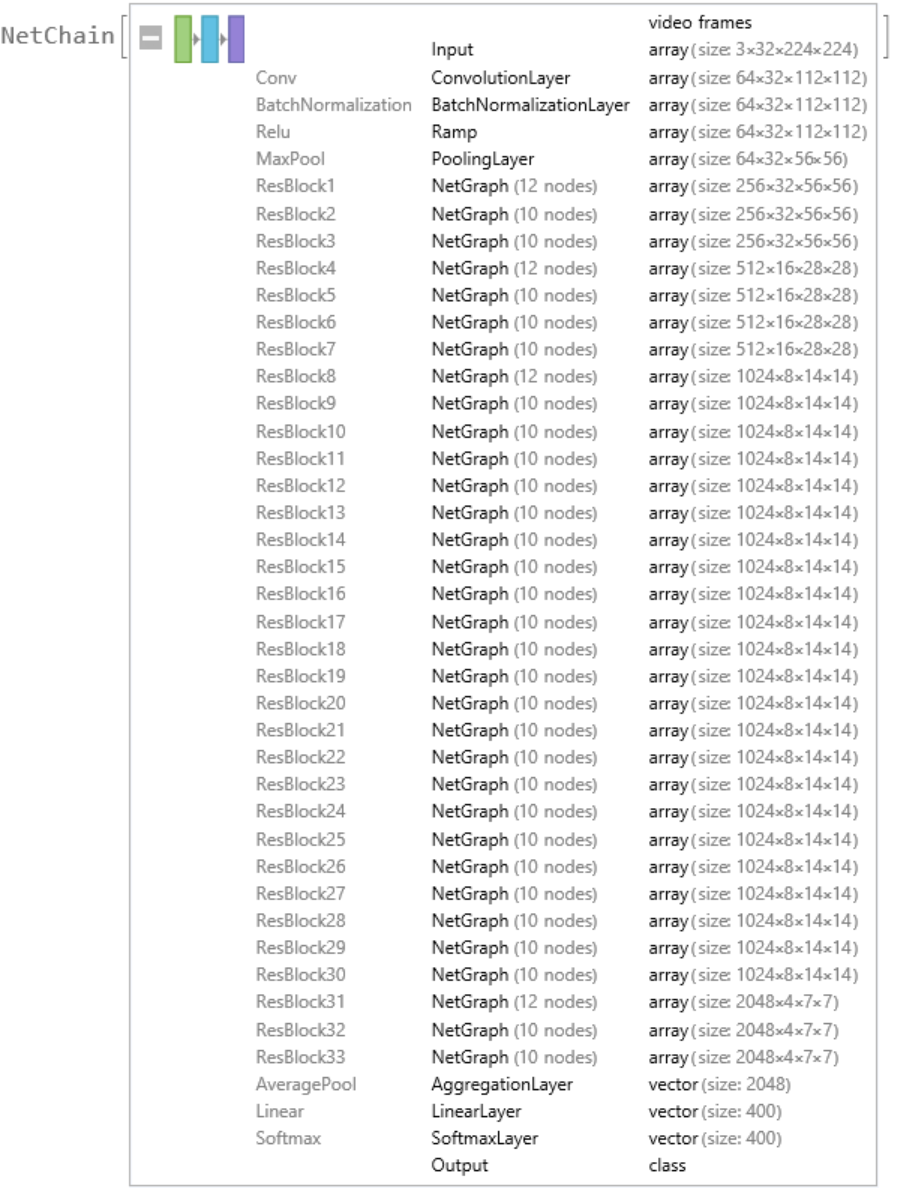### Basic usage

Classify a video:

 In:=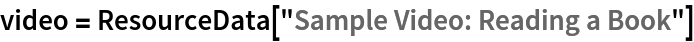Out=In:=Out=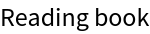Obtain the probabilities predicted by the net:

 In:=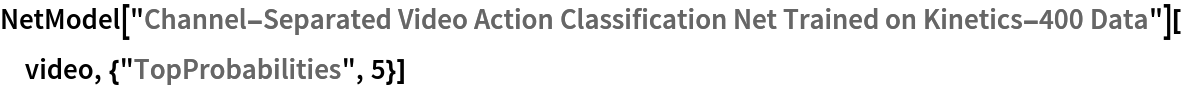Out=### Feature extraction

Remove the last three layers of the trained net so that the net produces a vector representation of an image:

 In:=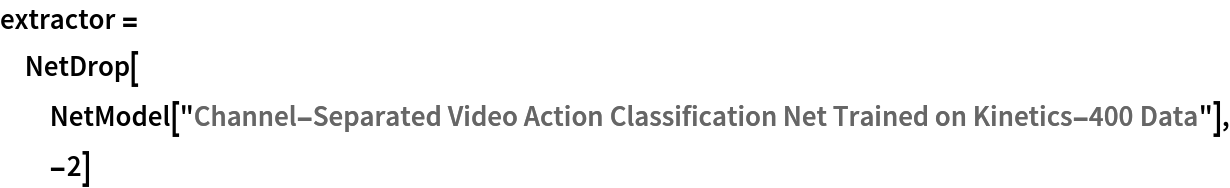Out=Get a set of videos:

 In:=Visualize the features of a set of videos:

 In:=Out=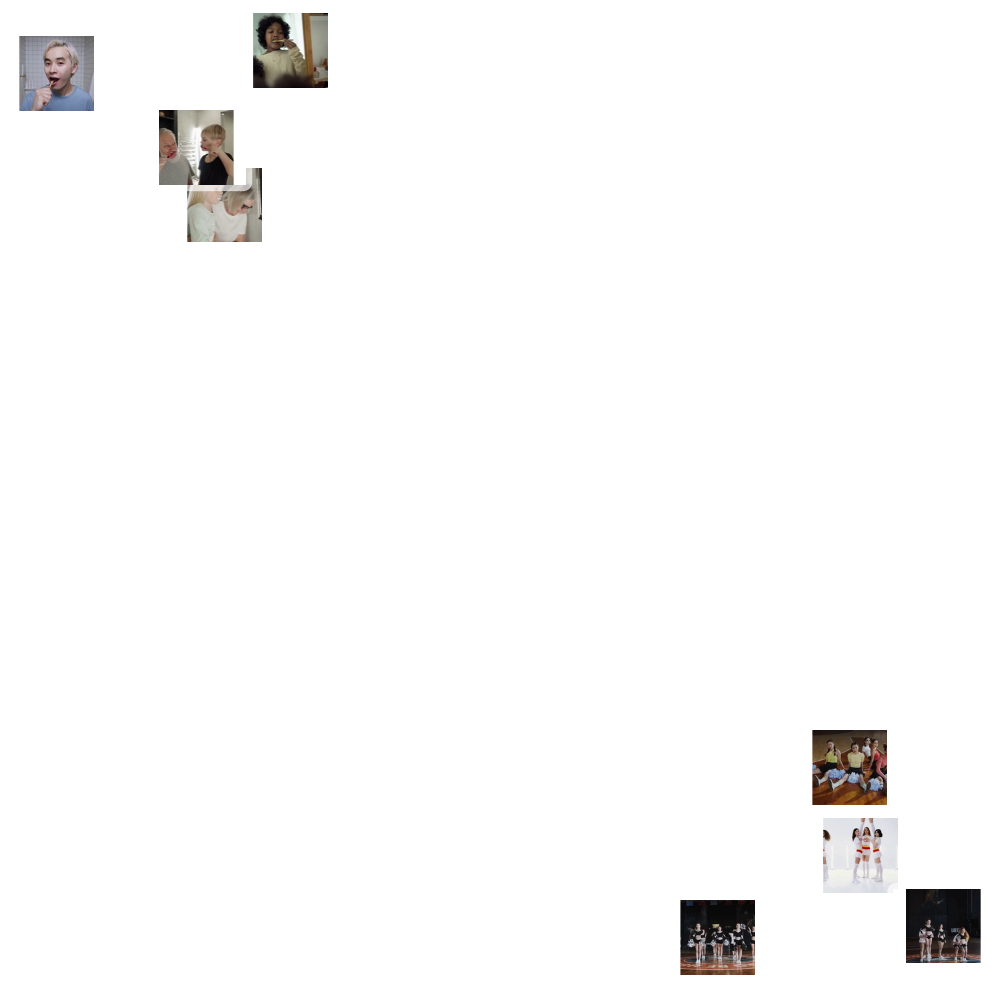### Transfer learning

Use the pre-trained model to build a classifier for telling apart images from two action classes not present in the dataset. Create a test set and a training set:

 In:=In:=In:=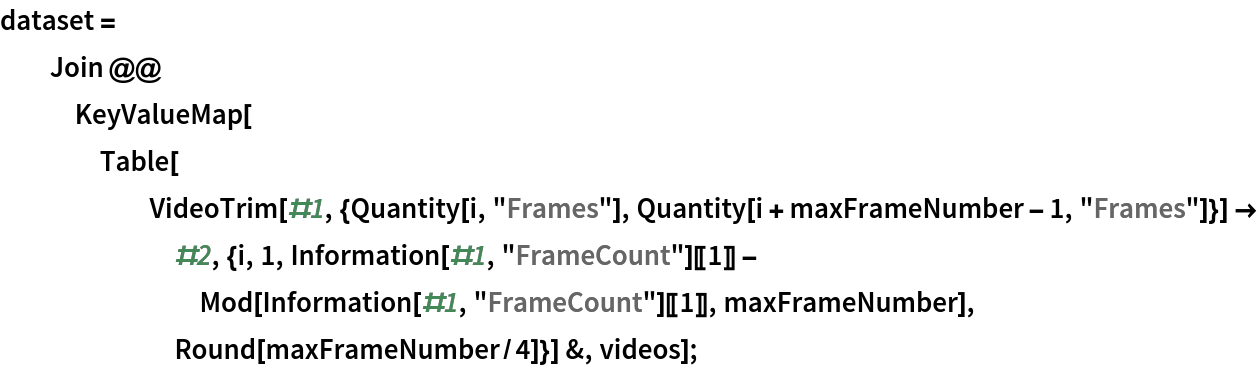In:=Remove the linear and the softmax layers from the pre-trained net:

 In:=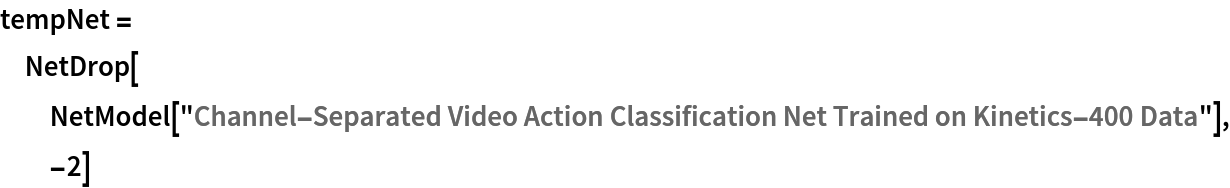Out=Create a new net composed of the pre-trained net followed by a LinearLayer and a SoftmaxLayer:

 In:=Out=Train on the dataset, freezing all the weights except for those in the "Linear" layer (use TargetDevice-> "GPU" for training on a GPU):

 In:=Out=Perfect accuracy is obtained on the test set:

 In:=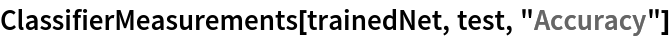Out=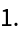### Net information

Inspect the number of parameters of all arrays in the net:

 In:=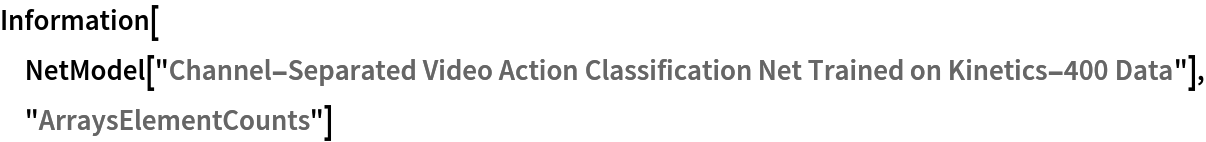Out=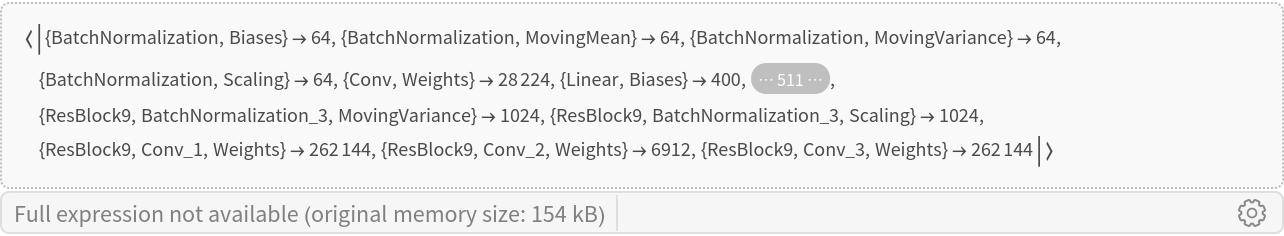Obtain the total number of parameters:

 In:=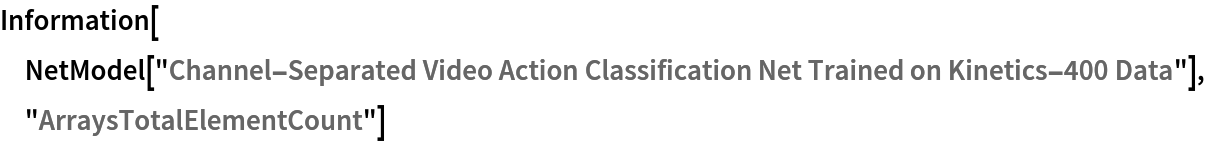Out=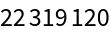Obtain the layer type counts:

 In:=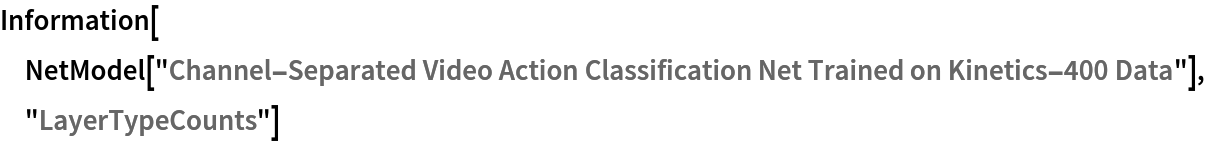Out=Display the summary graphic:

 In:=Out=